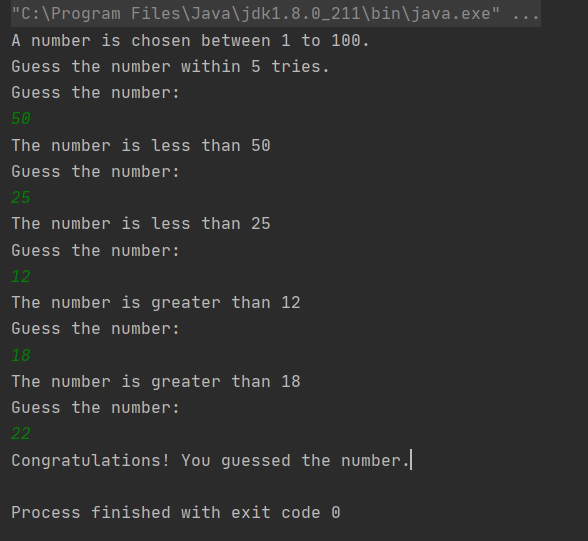# Number guessing game in Java

• Difficulty Level : Hard
• Last Updated : 05 Oct, 2021

The task is to write a Java program in which a user will get K trials to guess a randomly generated number. Below are the rules of the game:

• If the guessed number is bigger than the actual number, the program will respond with the message that the guessed number is higher than the actual number.
• If the guessed number is smaller than the actual number, the program will respond with the message that the guessed number is lower than the actual number.
• If the guessed number is equal to the actual number or if the K trials are exhausted, the program will end with a suitable message.

Approach: Below are the steps:

Attention reader! Don’t stop learning now. Get hold of all the important DSA concepts with the DSA Self Paced Course at a student-friendly price and become industry ready.  To complete your preparation from learning a language to DS Algo and many more,  please refer Complete Interview Preparation Course.

In case you wish to attend live classes with experts, please refer DSA Live Classes for Working Professionals and Competitive Programming Live for Students.

• The approach is to generate a random number using Math.random() method in Java.
• Now using a loop, take K input from the user and for each input print whether the number is smaller or larger than the actual number.
• If within K trials the user guessed the number correctly, print that the user won.
• Else print that he was not able to guess and then print the actual number.

Below is the implementation of the above approach:

## Java

 `// Java program for the above approach``import` `java.util.Scanner;` `public` `class` `GFG {` `    ``// Function that implements the``    ``// number guessing game``    ``public` `static` `void``    ``guessingNumberGame()``    ``{``        ``// Scanner Class``        ``Scanner sc = ``new` `Scanner(System.in);` `        ``// Generate the numbers``        ``int` `number = ``1` `+ (``int``)(``100``                               ``* Math.random());` `        ``// Given K trials``        ``int` `K = ``5``;` `        ``int` `i, guess;` `        ``System.out.println(``            ``"A number is chosen"``            ``+ ``" between 1 to 100."``            ``+ ``"Guess the number"``            ``+ ``" within 5 trials."``);` `        ``// Iterate over K Trials``        ``for` `(i = ``0``; i < K; i++) {` `            ``System.out.println(``                ``"Guess the number:"``);` `            ``// Take input for guessing``            ``guess = sc.nextInt();` `            ``// If the number is guessed``            ``if` `(number == guess) {``                ``System.out.println(``                    ``"Congratulations!"``                    ``+ ``" You guessed the number."``);``                ``break``;``            ``}``            ``else` `if` `(number > guess``                     ``&& i != K - ``1``) {``                ``System.out.println(``                    ``"The number is "``                    ``+ ``"greater than "` `+ guess);``            ``}``            ``else` `if` `(number < guess``                     ``&& i != K - ``1``) {``                ``System.out.println(``                    ``"The number is"``                    ``+ ``" less than "` `+ guess);``            ``}``        ``}` `        ``if` `(i == K) {``            ``System.out.println(``                ``"You have exhausted"``                ``+ ``" K trials."``);` `            ``System.out.println(``                ``"The number was "` `+ number);``        ``}``    ``}` `    ``// Driver Code``    ``public` `static` `void``    ``main(String arg[])``    ``{` `        ``// Function Call``        ``guessingNumberGame();``    ``}``}`

Output:

Below is the output of the above program:My Personal Notes arrow_drop_up